# Test: Basic Nodal & Mesh Analysis

## 10 Questions MCQ Test Topicwise Question Bank for Electrical Engineering | Test: Basic Nodal & Mesh Analysis

Description
Attempt Test: Basic Nodal & Mesh Analysis | 10 questions in 30 minutes | Mock test for Electrical Engineering (EE) preparation | Free important questions MCQ to study Topicwise Question Bank for Electrical Engineering for Electrical Engineering (EE) Exam | Download free PDF with solutions
QUESTION: 1

Solution:
QUESTION: 2

### Which of the following is ​​​​true about the circuit shown below?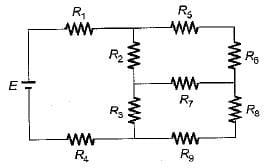Solution:

Number of junction point for the given circuit is 6.

QUESTION: 3

### For the circuit shown below, the current through the 1 Ω resistor is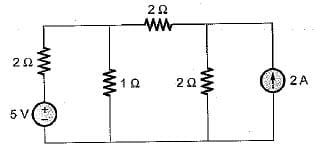Solution:

Using source transformation, the current source is converted to a voltage source as shown below.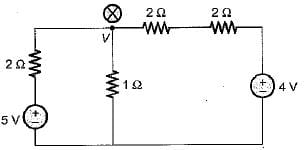Applying nodal analysis at node (x), we get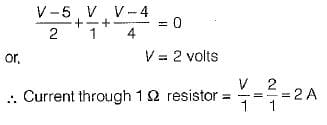QUESTION: 4

An electric circuit with 8 branches and 4 nodes will have

Solution:

Number of loop equation
= b - ( n - 1)
= 8 - ( 4 - 1 )
= 8 - 3 = 5

QUESTION: 5

For the circuit shown below, the current through the 10 V battery is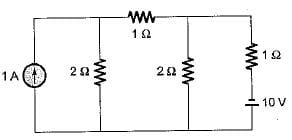Solution:

Using source transformation, first we convert the current source into an equivalent voltage source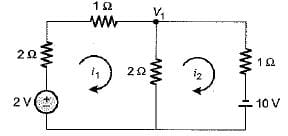Applying KVL in loop-1, we get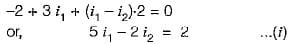Applying KVL in loop-2, we get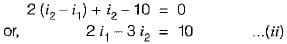On solving equations (i) and (//). we get

i1 = 2.36 A and i2 = 4.91 A

Hence, the current through the battery of 10 V is

i2 = 4.91 A

QUESTION: 6

Find the value of the currents I1 and I2 flowing clockwise in the first and second mesh respectively.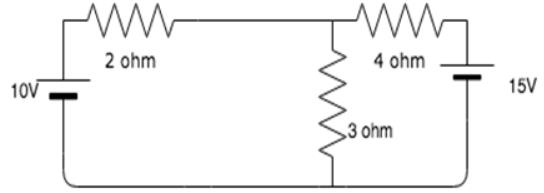Solution:

The two mesh equations are:
5I1 - 3I2 = 10
-3I1 + 7I2 = -15
Solving the equations simultaneously, we get I1 = 0.96A and I2 = -1.73A.

QUESTION: 7

The value of V in volts for the circuit shown below is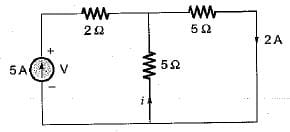Solution:

The equivalent resistance across the 5 A current source is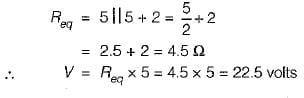QUESTION: 8

The number of KVL and KCL equations for the circuit shown below are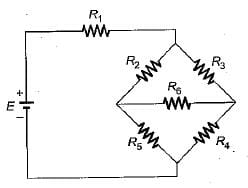Solution:

Number of nodes, n = 5
Number of branchs, b = 6
Hence, number of KCL equation
= n - 1 = 5 - 1 = 4
and, number of KVL equations = b - ( n - 1)
= 6 - (5-1) = 6- 4 = 2

QUESTION: 9

The current l flowing in the given figure is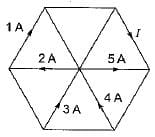Solution:
QUESTION: 10

The value of dependent source for the circuit shown below is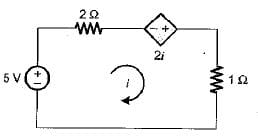Solution:

Applying KVL in the loop,
5 = 2i — 2i + i or i = 5 A
∴ Value of dependent source
= 2i = 2 x 5 = 10 voltUse Code STAYHOME200 and get INR 200 additional OFF Use Coupon Code